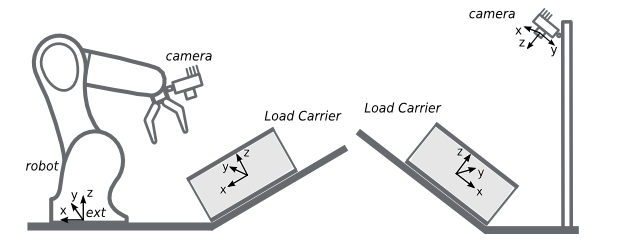# FAQs and troubleshooting¶

The load carrier rim is not visible in the depth image

One common case for missing the rim in depth images takes place when one of the edges of the load carrier is parallel to the baseline of the stereo system. It can be easily determined if this the case by slightly rotating the load carrier and observing if the orientation has an effect on the appearance of the object in the depth image. When this happens, the rc_randomdot projector can be added to the rc_visard to increase the depth image density.

The load carrier is not detected or not detected robustly

• Is the load carrier fully visible in both the left and right camera image?

• Does the load carrier rim appear in the depth image? (cfr. Detecting the load carrier)

• Is the load carrier inside the region of interest (if specified)?

The manufacturer’s dimensions might not be completely accurate. If the load carrier is not detected, we recommended to double check the configured dimensions. Additionally, one can try increasing the model_tolerance parameter (e.g. to the maximum value).

• The load carrier is not on a horizontal surface

• The load carrier is deformed

The load carrier is not placed on a horizontal surface

By default, the LoadCarrier module assumes that the load carrier is located on a horizontal surface. If that’s not the case, one needs to provide the load carrier orientation as a prior with the load carrier model. The orientation prior can be set in the Web GUI’s Modules ‣ LoadCarrier page and via the REST-API, which is described in the following.

Two sample cases are shown in Fig. 17 for the external pose frame (left) and the camera pose frame (right).Fig. 17 Load carrier not placed on a horizontal surface

In the left case of Fig. 17, the load carrier (tilted-load-carrier-ext) is rotated by an angle $$\theta$$ around the $$y$$ axis of the external coordinate system. The load carrier orientation is given by the following quaternion: $$\left[0, \sin(\theta/2), 0, \cos(\theta/2)\right]$$.

Request to the REST-API for configuring tilted-load-carrier-ext

Here we make the assumption that tilted-load-carrier-ext has the same dimensions of my-load-carrier-1 (cfr. Load carriers with a solid rim) and that the angle $$\theta$$ is 30 deg.

To trigger the set_load_carrier service via the REST-API for tilted-load-carrier-ext, one needs to send a PUT request to the URL http://<rc-visard-ip>/api/v2/nodes/rc_load_carrier_db/services/set_load_carrier, where <rc-visard-ip> should be replaced by the actual IP of the rc_visard.

The PUT body should include the following data, in JSON:

{
"args": {
"outer_dimensions": {
"x": 0.4,
"y": 0.3,
"z": 0.22
},
"inner_dimensions": {
"x": 0.37,
"y": 0.27,
"z": 0.215
},
"pose_frame": "external",
"pose": {
"orientation": {
"x": 0,
"y": 0.25882,
"z": 0,
"w": 0.96593
}
},
"pose_type": "ORIENTATION_PRIOR"
}
}
}


In the right case of Fig. 17, the load carrier (tilted-load-carrier-cam) is parallel to the image plane. Its orientation in the camera coordinate system is $$\left[\sqrt(2)/2, -\sqrt(2)/2, 0, 0\right]$$.

Request to the REST-API for configuring tilted-load-carrier-cam

Here we make the assumption that tilted-load-carrier-cam has the same dimensions of my-load-carrier-1 (cfr. Load carriers with a solid rim).

To trigger the set_load_carrier service via the REST-API for tilted-load-carrier-ext, one needs to send a PUT request to the URL http://<rc-visard-ip>/api/v2/nodes/rc_load_carrier_db/services/set_load_carrier, where <rc-visard-ip> should be replaced by the actual IP of the rc_visard.

The PUT body should include the following data, in JSON:

{
"args": {
"outer_dimensions": {
"x": 0.4,
"y": 0.3,
"z": 0.22
},
"inner_dimensions": {
"x": 0.37,
"y": 0.27,
"z": 0.215
},
"pose_frame": "camera",
"pose": {
"orientation": {
"x": 0.70711,
"y": -0.70711,
"z": 0,
"w": 0
}
},
"pose_type": "ORIENTATION_PRIOR"
}
}
}


One can try increasing the model_tolerance parameter (e.g. to the maximum value).

For significantly deformed load carriers, the detection algorithm might not provide reliable results. This can for example be the case of cardboard boxes after several uses. An alternative for such cases is to fix the load carrier placement and specify the exact pose of the load carrier (please see Setting the exact pose or an orientation prior of a load carrier for further discussion).

This means that either the load carrier $$z$$ inner dimension is too large or the reconstruction of the load carrier floor is noisy.

To improve the detection result as shown in Fig. 18, two options are available:

• Decrease the load carrier $$z$$ inner dimension
• Increase the crop_distace parameter (recommended for noisy data)

Objects on the load carrier floor are not detected as load carrier content

This means that either the load carrier $$z$$ inner dimension is too small or the the crop_distace parameter is too large.

There are multiple load carriers of the same type in the scene

In the current implementation, the LoadCarrier module detects one load carrier with each detect_load_carrier or detect_filling_level request. Similarly, the rc_reason modules using a load carrier can only detect a single load carrier.

If there are multiple load carriers of the same type in the scene, we recommended to specify one ore more regions of interest, each one including one load carrier instance.

My load carrier doesn’t move. How do I speed up my application?

One can reduce the estimation time for grasp points or object detections inside the load carrier by avoiding repeated load-carrier detection over multiple frames. The pose of the load carrier can be estimated once and then set as an exact pose. Subsequent grasp point computations or object detections use this exact pose, without requiring any load carrier detection anymore. Please see Setting the exact pose or an orientation prior of a load carrier for further discussion.